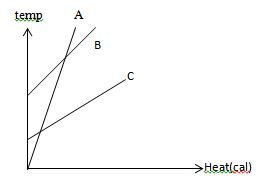# Specific heat capacity

Specific heat capacity is the amount of heat energy required to raise the temperature of a body per unit of mass. he specific heat is the amount of heat per unit mass required to raise thetemperature by one degree Celsius. The relationship between heat and temperature change is usually expressed in the form shown below where c is the specific heat. The relationship does not apply if a phase change is encountered, because the heat added or removed during a phase change does not change the temperature.

Q = cmΔT
Where
•